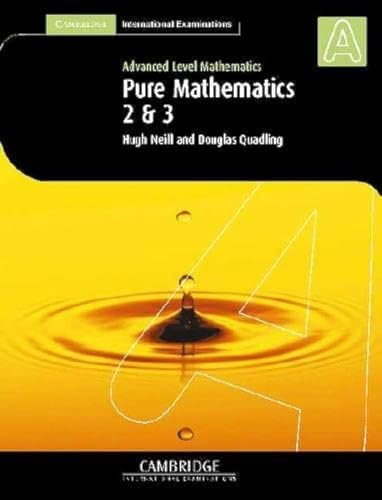# Pure Mathematics 2 and 3 (International) by Hugh Neill

In Stock
\$11.99
+10% GST
Pure Mathematics 2 corresponds to units P2 and P3. It covers algebra, logarithmic and exponential functions, trigonometry, differentiation, integration, numerical solution of equations, vectors, differential equations and complex numbers.
Only 2 left

## Pure Mathematics 2 and 3 (International) Summary

### Pure Mathematics 2 and 3 (International) by Hugh Neill

Written to match the contents of the Cambridge syllabus. Pure Mathematics 2 corresponds to units P2 and P3. It covers algebra, logarithmic and exponential functions, trigonometry, differentiation, integration, numerical solution of equations, vectors, differential equations and complex numbers.

### Why buy from World of BooksOur excellent value books literally don't cost the earthFree delivery in AustraliaEvery used book bought is one saved from landfill

Introduction; 1. The motion of projectiles; 2. Moments; 3. Centre of mass; 4. Rigid objects in equilibrium; 5. Elastic strings and springs; Revision exercise 1; 6. Motion round a circle; 7. Geometrical methods; 8. Centres of mass of special shapes; 9. Linear motion with variable forces; 10. Strategies for solving problems; Revision exercise 2; Practice examinations; Answers; Index; Formulae

GOR003602944
Pure Mathematics 2 and 3 (International) by Hugh Neill
Hugh Neill
Used - Very Good
Paperback
Cambridge University Press
2002-09-19
335
0521530121
9780521530125
N/A
Book picture is for illustrative purposes only, actual binding, cover or edition may vary.
This is a used book - there is no escaping the fact it has been read by someone else and it will show signs of wear and previous use. Overall we expect it to be in very good condition, but if you are not entirely satisfied please get in touch with us.